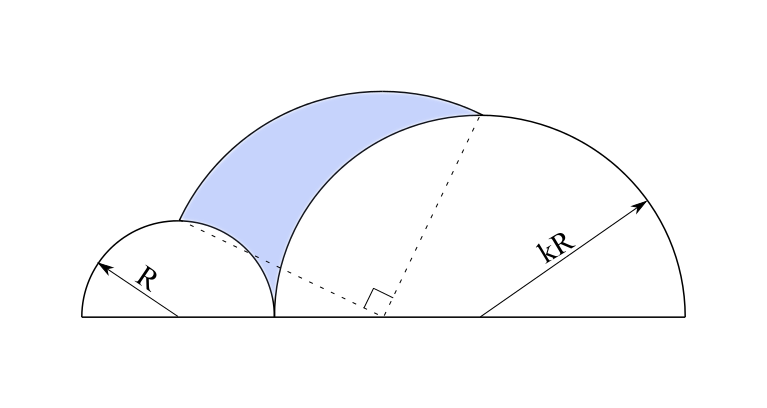# Semi-semicircle

Geometry Level 4Take a semicircle of radius $R$ and one semicircle $k$ times bigger and make them tangent like in the picture above. Then construct a ${{90}^{\circ }}$ arc through the midpoints of these 2 semicircles. If the shaded area is $A$, calculate the ratio $\dfrac{A}{kR^2}$.

Hint: Notice that all three centers are collinear.

×